# Distribution Difference of Two Independent Random Variables

## Homework Statement

Z = X - Y and I'm trying to find the PDF of Z.

Convolution

## The Attempt at a Solution

Started by finding the CDF:
Fz(z) = P(Z ≤ z)

P(X - Y ≤ z)

So I drew a picture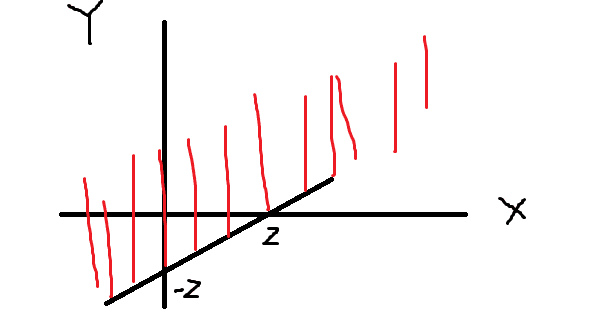So then should Fz(z) be: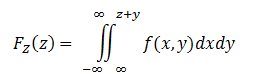since, from my graph, it looks as though Y can go from negative infinity to positive infinity, and X can go from negative infinity on the left but is bounded by z+y on the right

then should the pdf be:
Edit: sorry I forgot to include the fact that, because fx and fy are independent, f(x,y) = fxfy in the following derivation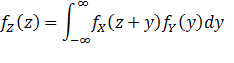?

Last edited:

Ray Vickson
Homework Helper
Dearly Missed

## Homework Statement

Z = X - Y and I'm trying to find the PDF of Z.

Convolution

## The Attempt at a Solution

Started by finding the CDF:
Fz(z) = P(Z ≤ z)

P(X - Y ≤ z)

So I drew a picture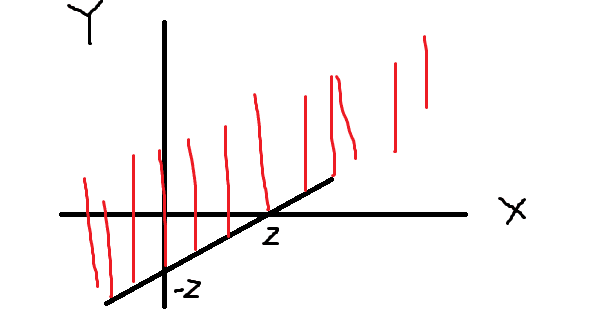So then should Fz(z) be: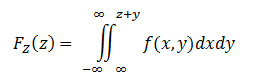since, from my graph, it looks as though Y can go from negative infinity to positive infinity, and X can go from negative infinity on the left but is bounded by z+y on the right

then should the pdf be:
Edit: sorry I forgot to include the fact that, because fx and fy are independent, f(x,y) = fxfy in the following derivation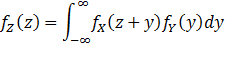?

Orodruin
Staff Emeritus
Homework Helper
Gold Member
Your approach looks reasonable. Is there something you are particularly worried about?

Oh yes, sorry I was wondering if what I arrived at for the PDF of the difference of two independent random variables was correct.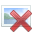I tried Googling but all I could find was the pdf of the sum of two RVs, which I know how to do already.

Orodruin
Staff Emeritus
Homework Helper
Gold Member
Yes, it looks ok. You should note that if you know the pdf of the sum and the pdf of the negative of a distribution (i.e., if you know how the the distribution of ##Y##, what is the distribution of ##-Y##), then you can use these two to obtain the distribution of ##X-Y##.

•izelkay
Yes, it looks ok. You should note that if you know the pdf of the sum and the pdf of the negative of a distribution (i.e., if you know how the the distribution of ##Y##, what is the distribution of ##-Y##), then you can use these two to obtain the distribution of ##X-Y##.
I'm going to modify the problem a little bit, can you tell me if I do it right/wrong?

X and Y are two independent random variables, each of which are uniform on (0,1). Find the pdf of Z = X - Y

Using my result from above, the pdf of Z is given by: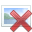X ~ U(0,1) and Y ~ U(0,1)

The pdf of fx(x) and fY(y) are both 1 on (0,1) and 0 otherwise.

z goes from -1 to 1 because of X - Y right?

For -1 ≤ z ≤ 0
fy is uniform only on (0,1) so my integral limits should be z+1 to z

For 0 ≤ z ≤ 1 I wouldn't need to change the limits here, just from 0 to 1

So then the pdf of X - Y is: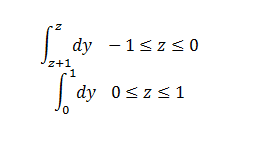Is this correct?

Ray Vickson
Homework Helper
Dearly Missed
I'm going to modify the problem a little bit, can you tell me if I do it right/wrong?

X and Y are two independent random variables, each of which are uniform on (0,1). Find the pdf of Z = X - Y

Using my result from above, the pdf of Z is given by:X ~ U(0,1) and Y ~ U(0,1)

The pdf of fx(x) and fY(y) are both 1 on (0,1) and 0 otherwise.

z goes from -1 to 1 because of X - Y right?

For -1 ≤ z ≤ 0
fy is uniform only on (0,1) so my integral limits should be z+1 to z

For 0 ≤ z ≤ 1 I wouldn't need to change the limits here, just from 0 to 1

So then the pdf of X - Y is: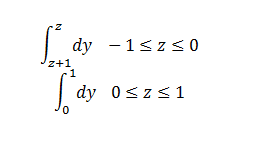Is this correct?

No, it cannot possibly be correct, because you would have f(z) = -1 for -1 < z < 0 and f(z) = 1 for 0 < z < 1. That kind of f cannot be a probability density function.

You need to go back and evaluate the limits more carefully; in cases like this, drawing an x,y diagram of the integration region would be helpful.

•izelkay
No, it cannot possibly be correct, because you would have f(z) = -1 for -1 < z < 0 and f(z) = 1 for 0 < z < 1. That kind of f cannot be a probability density function.

You need to go back and evaluate the limits more carefully; in cases like this, drawing an x,y diagram of the integration region would be helpful.
I don't have much practice drawing integration regions of density functions and I wouldn't know where to start with this one besides drawing the box with vertices (0,0), (0,1), (1,0), (1,1).

I think I found another way to look at it though:

If -1 ≤ z ≤ 0,

If I want fx(z+y) to be 1, I need to shift the bounds by +1, and then I'll need z+y+1 ≥ 0 , or y ≥ -1-z

So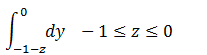Then on 0 ≤ z ≤ 1,

I need z+y ≤ 1, or y ≤ 1 - z

So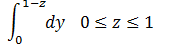Then all together this is:

1 + z , -1 ≤ z ≤ 0
1 - z , 0 ≤ z ≤ 1

I got the same answer as here: http://www.math.wm.edu/~leemis/chart/UDR/PDFs/StandarduniformStandardtriangular.pdf
but I'm not sure my process is correct because my bounds are a little different than theirs, and I don't know how they computed the cdf

Ray Vickson
Homework Helper
Dearly Missed
I don't have much practice drawing integration regions of density functions and I wouldn't know where to start with this one besides drawing the box with vertices (0,0), (0,1), (1,0), (1,1).

I think I found another way to look at it though:

If -1 ≤ z ≤ 0,

If I want fx(z+y) to be 1, I need to shift the bounds by +1, and then I'll need z+y+1 ≥ 0 , or y ≥ -1-z

SoThen on 0 ≤ z ≤ 1,

I need z+y ≤ 1, or y ≤ 1 - z

So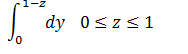Then all together this is:

1 + z , -1 ≤ z ≤ 0
1 - z , 0 ≤ z ≤ 1

I got the same answer as here: http://www.math.wm.edu/~leemis/chart/UDR/PDFs/StandarduniformStandardtriangular.pdf
but I'm not sure my process is correct because my bounds are a little different than theirs, and I don't know how they computed the cdf

You already drew part of the diagram in Post #1. You just need to add the boundary lines x = 0, x = 1, y = 0, y = 1 and you are done---that's your drawing!

You say you "don't know how they computed the cdf". Well, you claimed before that you know how to compute the cdf of a SUM, and that is all you need to do.

If ##Z = X-Y## then ##S = Z+1## has the form ##S = X + (1-Y) = X + Y'##, where ##X## and ##Y' = 1-Y## are independent and ##\rm{U}(0,1)## random variables. Thus, ##S## is the sum of two uniforms, and has a well-known, widely-documented triangular density:
$$f_S(s) = \begin{cases} s, & 0 < s < 1\\ 2-s, & 1 < s < 2\\ 0 & \rm{elsewhere} \end{cases}$$
Now just shift the graph of ##f_S(s)## one unit to the left, and that would be your density of ##Z##: ##f_Z(z) = f_S(1+z), \; -1 < z < 1##.

Last edited:
•izelkay